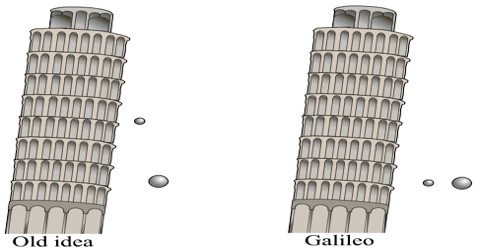# Galileo’s three laws about Falling Bodies

Galileo’s three laws about Falling Bodies

We often see that when a heavy body and a light body are allowed to fall from the same height, the heavy body reaches the ground earlier than the light body. Since acceleration due to gravity acting on a body does not depend on the mass of the body, so the acceleration due to gravity acting on heavy and light bodies will be the same. So they are supposed to reach the ground at the same time. The difference in time found in falling to the ground is due to the air resistance. Galileo allowed falling different bodies having different masses from the top of the high observatory and showed that they reached the ground almost at the same time. If there would have no air hindrance they would have reached the ground exactly at the same time. As the two bodies remain in the air, so buoyancy of air acts opposite to their weight. The buoyancy or upward force is more on the lighter body than the heavier ones, so the lighter body reaches late than the heavier body. Since acceleration due to gravity acting on a body does not depend on the mass of the body, so acceleration due to gravity acting on the heavy and light bodies is the same.

Galileo (1564-1642) was the first to determine, at the start of the seventeenth century, the law of constant acceleration of free-falling bodies. Galileo gave three laws about falling bodies. These are called Galileo’s laws in the case of falling bodies. These Laws are applicable to freely falling bodies. The law states that the distances traveled are proportional to the squares of the elapsed times.

Laws are given below:

First law: In a vacuum, all the freely falling bodies starting from rest traverse equal distance at equal interval of time or, in a vacuum all bodies starting from rest fall with equal rapidity.

Explanation: According to this law if different objects having different sizes, namely volumes are allowed to fall from rest at a particular height and without any resistance will reach the ground at the same time. The first law can be proved by the guinea and feather experiment.

Second law: Starling from rest, the velocity of a freely falling body is proportional to the time taken to fall. If a falling body gets velocity v at time t, it can be mathematically written as, v ∞ t.

Explanation: If a body is allowed to fall freely from rest under the action of gravitational force, then if its velocity in one second is v, then the velocity in two seconds will be 2v, in three seconds 3v and so on. Generally, if the velocities of the falling body in t1, t2, t3 secs, are respectively v1, v2, v3 then we find,

v1/t1 = v2/t2 = v3/t3

So, v ∞ t

Third law: Starting from rest, the distance traversed by a freely falling body is proportional to the square of the time of fall. If a falling body traverses distance h in time t, it can be mathematically written as, h ∞ t2.

Explanation: Under gravitational attraction, if a body, initially at rest, is allowed to fall freely then the body will traverse distance h in 1 sec., distance 22 x h in 2 sec., 32 x h in 3 sec. and so on. In other words, in equal successive periods of time, the distances traveled by a free-falling body are proportional to the succession of odd numbers (1, 3, 5, 7, etc.). So if the body traverses distances h1, h2, and h respectively, in t1, t2, t3 secs., then,

h1/t12 = h2/t22 = h3/t32

so, h ∞ t2.